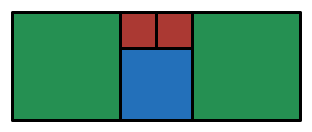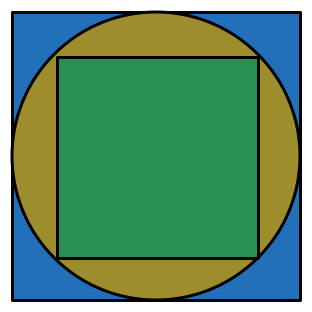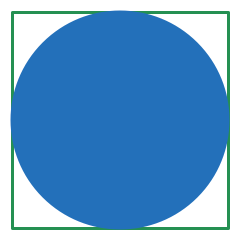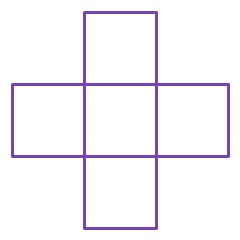Geometry

# Length and Area WarmupThe above image shows a collection of squares. If each red square has a side length of 1 unit, what is the area of the entire figure?In the figure above, a red cross is formed by placing two $7 \times 5$ rectangles over each other. What is the perimeter of the figure?Within a large square, we inscribe a circle.
This circle circumscribes another square.

What is the ratio of the area of the smaller square to the larger square?If the perimeter of the square is 56, what is the area of the circle contained within?If each individual square has a side length of 3, what is the perimeter of the cross?

×